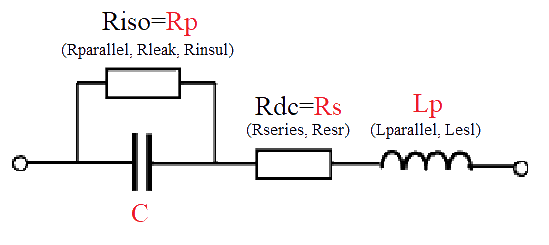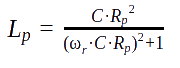# How to compare and cross-reference Panasonic capacitors datasheet to other electrolytic capacitor manufacturers?

## A model for Panasonic aluminum electrolytic capacitors ESRj

The model takes a selection of different Panasonic series and uses measured parts of training data for a given number of Panasonic electrolytic capacitors. Next it creates a polynomial expression at each frequency of interest. For a series of capacitors measured over different ranges of frequencies, two different cases are handled.
It then aggregates 10 different samples in order to provide a stable set of results, which are reliable and can be used for accurate curve prediction. Lab measurements are provided from a Certified Lab, conducting EMI measurements and tests according to.

## Details on impedance vs. frequency lab measured simulations

The frequency range of interest is created with corresponding values and a curve fit is carried out at each frequency (inner polynomial). The parameters generated from this curve-fit are then fitted again (outer polynomial) to enable a flexible horizontal axis.
In order to accommodate the large range of capacitance and frequencies encountered in the series, the model applies logarithmic and exponential functions as required. This has greatly improved the prediction results as tested on various types of capacitors and MLCC dielectrics.

## Example series of Panasonic capacitors: SXVOS-CON™, SXV ,SXEOS-CON™, SXE, SVPCOS-CON™, SVPC, SVPDOS-CON™, SVPD,

### Calculations and used formulas

Key:
• Z - Impedance
• Zeq - Equivalent impedance
• |Z|min - min value of |Z|
• R - Resistance
• Rs - parasitic resistance in series
• Rp = Riso - parasitic resistance in parallel, isolation resistance
• L - Inductance
• Lp - parasitic inductance
• C - Capacitance
• F - Frequency
• Fres / SRF - the self resonant frequency
• ω - Angular frequency
• ω_res / ω_r / ω_srf - the self resonant frequency
• ω = 2*π*F
Frequency range:
F axis calibration in case of different F ranges in the same series:
Few cases (so far):
• F range_#1: 0.00002 M[Hz] - 120 M[Hz]
• F range_#2: 0.001 M[Hz] - 120 M[Hz]
• F range_#3: 1 M[Hz] - 3000 M[Hz]
Check the distance between the SRF to the median of each of the F ranges in the input and the fit the closest range.
e.g. 200 steps, median index = 100
With the relevant measured dependencies for each cap:
The capacitor equivalent circuit:Calculate the Z by the next formulas for each cap:
Calculated parameters, based on the inputs:The impedance frequency response, based on the parameters:## Summary and conclusion

The measurements of enough samples and the model together result in a precise data pool of the main capacitor parameters for a given series. In addition, this enables also a projection of parameters for un-measured products within a series resulting in a complete picture of a selected Panasonic capacitor series. With enough measured samples a full range/portfolio of capacitors can be represented.
Try and use the parametric search for Panasonic capacitors. Looking for another manufacturer, please click here for a fresh parametric search.